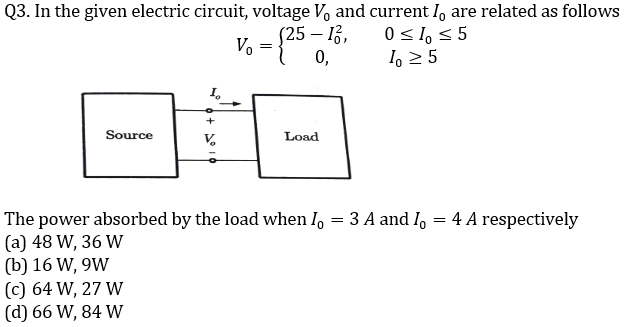Engineering Jobs   »   Electrical Engineering quizs   »   GATE 2022, ELECTRICAL GATE QUIZ,

# GATE’22 EE: Weekly Practices Quiz 25-Sep-2021

## GATE 2022 Important Dates

Organizing Institute for GATE 2022 is the Indian Institute of Technology Kharagpur. For GATE 2022 Exam IIT Kharagpur has released the official notification and Important dates regarding the GATE 2022 Exam. Aspirants preparing for GATE 2022 must check the details regarding GATE 2022 Important Dates.

## GATE’22 EE:  Practices Quiz

GATE’22 EE: Weekly Practices Quiz 25-Sep-2021

Each question carries 2 marks
Negative marking: 2/3 mark

Total Questions: 10
Total marks: 20
Time: 20 min.

Q1. A 230 V dc step down chopper is driving a load resistance of 10 Ω. It has a voltage drop of 2 V across chopper when it is on. Find the rms value of output voltage for a duty cycle of 0.4.
(a) 0.2 × 228 V
(b) 0.2 × 230 V
(c) √(0.4 )× 230 V
(d) √0.4 × 228 V

Q2. A step-down chopper has Vs = 230 V and R = 10 Ω. For a duty cycle of 0.4, the power taken by the chopper is 2097 Watts. Find the chopper efficiency. Take the voltage drop across the chopper switch as 2 V.
(a) 98 %
(b) 89.96 %
(c) 99.14 %
(d) 96.54 %Q4. The voltage applied to a transformer primary is increased keeping V/f fixed. How will core loss and magnetizing current change?
(a) Core loss will increase and magnetizing current remain same
(b) Core loss will remain same and magnetizing current will remain same
(c) Core loss will decrease and magnetizing current will increase
(d) Core loss will remain same and magnetizing current will decrease

Q5. A dc shunt motor is running at 1000 rpm at the rated load torque. If few of the field windings get shot circuited then
(a) motor speed will increase and more armature current is drawn
(b) motor speed will decrease and more armature current is drawn
(c) motor speed will increase and lesser armature current is drawn
(d) motor speed will decrease and lesser armature current is drawn

Q6. For the equation, s^3-4s^2+s+6=0 the number of roots in the left half of s-plane will be
(a) Zero
(b) One
(c) Two
(d) Three

Q7. In a Q-meter measurement to determine the self-capacitance of a coil, the first resonance occurred at f_1 with C_1=300 pF. The second resonance occurred at f_2=2f1 with C_2=60 pF. The self-capacitance of coil works out to be:
(a) 240 pF
(b) 20 pF
(c) 360 pF
(d) 60 pF

Q8. An n-channel JFET having a pinch-off voltage (Vp) of -5 V shows a transconductance (g_m) of 1 mA/V, when the applied Gate to source (V_GS) is -3 V. Its maximum transconductance (in mA/V) is:
(a) 1.5
(b) 2.0
(c) 2.5
(d) 3.0

Q9. What is the value of charging current in short transmission lines?
(a) Less than medium transmission lines
(b) Equal to medium transmission lines
(c) More than medium transmission lines
(d) More than long transmission lines

Q10. In a three-phase induction motor, the ratio of starting current to full load current is 12.56 and the ratio of starting torque to full load torque is 1.6. percentage slip at full load is………
(a) 0.1 %
(b) 1%
(c) 12.56 %
(d) None of the above

## SOLUTIONS

S1. Ans.(d)
Sol. The voltage drop across the switch will occurs only when it is ON.
∴V_0 (rms)=√D (V_s-V_d )
⇒V_0 (rms)=√0.4 (230-2)=√0.4 × 228 V

S2. Ans.(c)
Sol. V_o (rms) = √(0.4 )× (230 – 2) = 144.2 V
P_o = 144.22/10 = 2079.3 Watts
P_i = 2097 Watts
∴η = Po/Pi = 99.14 %.

S3. Ans.(a)
Sol. For, 0≤I_0≤5
Power absorbed(P_0 )=V_0 I_0=(25-I_0^2 ) I_0
For I_0=3 A,P_01=(25-3^2 )×3=48 W
For I_0=4 A,P_01=(25-4^2 )×4=36 W

S4. Ans.(a)
Sol. In transformer, the magnetizing current I_m α V/f. If V/f is kept constant then magnetizing current will remain same and core loss depends on frequency. So, it will increase with increase in frequency.
As V/f is constant.
∴Wh α f & W(e ) α f^2
As voltage is increasing by keeping V/f ratio constant i.e., frequency also increased. Therefore, both hysteresis and eddy current losses will increase, and hence core losses will increase.

S5. Ans.(a)
Sol. If field winding gets short circuited, field excitation reduces causing rise in speed and more armature current.

S6. Ans.(b)
Sol. s^3-4s^2+s+6=0
by R-H criterion:

 s^3 1 1 s^2 -4 6 s^1 s^0 6

Here there is two sign changes( s^3 to s^2 and s^2 to s^1).
So, number of roots in RHS=2
∴Number of roots in LHS=3-2=1.

S7. Ans.(b)
Sol. Self-capacitance=(C1-4C2)/3=(300-(4×60))/3=60/3=20 pF

S8. Ans.(c)
Sol. g_m=-(2 IDSS)/V_p (1-VGS/Vp )
∴1 mA/V=-(2IDSS)/(-5)(1-(-3)/(-5))
⇒IDSS=(5/2)^2 mA
∴(gm )max=-(2 IDSS)/Vp =-2/(-5)×25/4=2.5 mA/V

S9. Ans.(a)
Sol. Line to Earth capacitance of short transmission line is less than that in medium and long transmission line and is negligible. Because of this the charging current of short transmission line is less than that in medium and long transmission line and is negligible.

S10. Ans.(b)
Sol. In a three-phase induction motor, Tst/Tfl =(Ist/Ifl )^2 sfl
∴1.6=(12.56)^2 sfl
∴sfl=0.01 or 1%

Sharing is caring!

Thank You, Your details have been submitted we will get back to you.